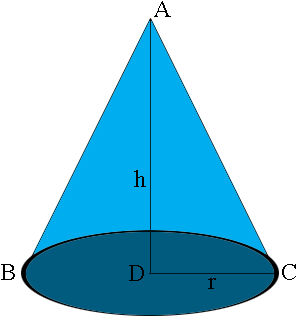SEARCH HOMEMath Central Quandaries & QueriesQuestion from John, a parent: Hi my daughter came with a seemingly easy question (which to me it was not) How to make/calculate a cone of a specific angle from top to bottom radius.Hi John,

To describe a cone we usually use the height $h$ and the radius of the base $r.$What you want is to guarantee that when the cone is constructed the measure of the angle |CAB| is specific number, call it $t$ degrees. The measure of the angle $CAD$ is then $\large \frac{t}{2}$ degrees. What is the relationship between the measure of the angle $CAD$ and the lengths $r$ and $h?$

I hope this helps,
PennyMath Central is supported by the University of Regina and The Pacific Institute for the Mathematical Sciences.